# How to use the noise calculator

## Simple sound pressure level calculations.

Insert information into the relevant cells, for example if you know what the sound pressure level was at a known distance then add these two figures in the relevant cells and click on the cell you want the answer i.e. the sound power level. Now you can change the distance to see what the resultant noise level will be at that new distance or some other location. Once the new distance is inserted click the level again to see the results in terms of the sound pressure level.

If you have a sound power level then this can be input along with the distance to the receiver find out the sound pressure level at that distance.

## Adding sources of noise together.

The table allows you to input three different noise sources that are at different distances from the receiver location and calculate the resultant noise at that location. It also allows you to determine the average noise over any period of time by setting the percentage on-time in that time period for each source.

## Adding noise sources measured at a receiver location.

Where you just want to find the effect of adding noise sources together at a receiver location, to find the resultant level then choose 1 metre distant and input the sound pressure level. Click the box on the combine sources frame and they will each be added together.

This noise calculator does not take into account any screening features, effects of the atmosphere or wind speed / direction.

## Directivity.

If you place a source of noise against a wall then more of the sound is directed away from the wall. Where this happens, simple calculations may under-estimate the noise level and the Source directivity (Q) part enables a correction to be made.

By default, all sources are treated in the same way and adjusted for directivity. This can be over-ridden by un-ticking the check box labelled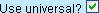. The universal adjustment for directivity is marked in green: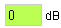To easily assess the chosen directivity, each source has an image illustrating the space of propagation.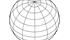### Whole space (Q = 1)

Where there are no reflections, for example over soft ground. This is the most common case for sound propagation and if in doubt, use this one.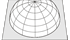### Half space (Q = 2)

Where the sound is being reflected off one surface, for example a source over hard ground. In this case an adjustment of 3dB is used, as the combination of two equal sounds equals a 3dB increase.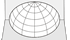### Quarter space (Q = 4)

For example, where a source is above hard ground at the foot of a wall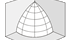### Eighth space (Q = 8)

For example, where the source is in the corner of a room.